# Balbharati solutions for Maharashtra state board (SSC) 10th Standard Maths 2 Geometry chapter 7 - Mensuration [Latest edition]

#### Chapters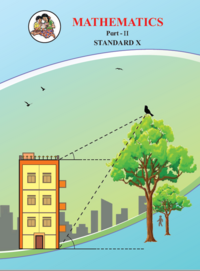## Chapter 7: Mensuration

Practice set 7.1Practice set 7.2Practice set 7.3Practice set 7.4
Practice set 7.1 [Pages 145 - 146]

### Balbharati solutions for Maharashtra state board (SSC) 10th Standard Maths 2 Geometry Chapter 7 Mensuration Practice set 7.1 [Pages 145 - 146]

Practice set 7.1 | Q 1 | Page 145

Find the volume of a cone if the radius of its base is 1.5 cm and its perpendicular height is 5 cm.

Practice set 7.1 | Q 2 | Page 145

Find the volume of a sphere of diameter 6 cm.

Practice set 7.1 | Q 3 | Page 145

Find the total surface area of a cylinder if the radius of its base is 5 cm and height is 40 cm.

Practice set 7.1 | Q 4 | Page 145

Find the surface area of a sphere of radius 7 cm.

Practice set 7.1 | Q 5 | Page 145

The dimensions of a cuboid are 44 cm, 21 cm, 12 cm. It is melted and a cone of height 24 cm is made. Find the radius of its base.

Practice set 7.1 | Q 6 | Page 145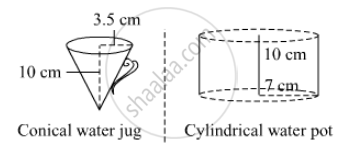Observe the measures of pots In the given figure. How many jugs of water can the cylindrical pot hold?

Practice set 7.1 | Q 7 | Page 145

A cylinder and a cone have equal bases. The height of the cylinder is 3 cm and the area of its base is 100 cm2. The cone is placed
upon the cylinder. The volume of the solid figure so formed is 500 cm3. Find the total height of the figure.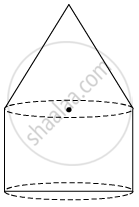Practice set 7.1 | Q 8 | Page 145

In the given figure, a toy made from a hemisphere, a cylinder and a cone are shown. Find the total area of the toy.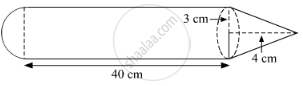Practice set 7.1 | Q 9 | Page 145

In the given figure, a cylindrical wrapper of flat tablets is shown. The radius of a tablet is 7 mm and its thickness is 5 mm. How many such tablets are wrapped in the wrapper?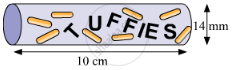Practice set 7.1 | Q 10 | Page 145

In the given figure shows a toy. Its lower part is a hemisphere and the upper part is a cone. Find the volume and surface area of the toy from the measures shown in the figure ($\pi = 3 . 14$)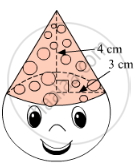Practice set 7.1 | Q 11 | Page 146

Find the surface area and the volume of a beach ball shown in the figure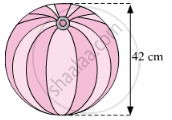Practice set 7.1 | Q 12 | Page 146

As shown in the figure, a cylindrical glass contains water. A metal sphere of diameter 2 cm is immersed in it. Find the volume of the water.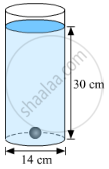Practice set 7.2 [Page 148]

### Balbharati solutions for Maharashtra state board (SSC) 10th Standard Maths 2 Geometry Chapter 7 Mensuration Practice set 7.2 [Page 148]

Practice set 7.2 | Q 1 | Page 148
The radii of two circular ends of a frustum shape bucket are 14 cm and 7 cm. The height of the bucket is 30 cm. How many liters of water it can hold?
(1 litre = 1000 cm)
Practice set 7.2 | Q 2.1 | Page 148

The radii of ends of a frustum are 14 cm and 6 cm respectively and its height is 6 cm. Find its  curved surface area

Practice set 7.2 | Q 2.2 | Page 148

The radii of ends of a frustum are 14 cm and 6 cm respectively and its height is 6 cm. Find its total surface area

Practice set 7.2 | Q 2.3 | Page 148

The radii of ends of a frustum are 14 cm and 6 cm respectively and its height is 6 cm. Find its volume $\pi$ = 3.14)

Practice set 7.2 | Q 3 | Page 148

The circumferences of circular faces of a frustum are 132 cm and 88 cm and its height is 24 cm. To find the curved surface area of the frustum complete the following activity.( $\pi = \frac{22}{7}$)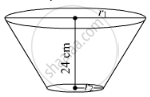Practice set 7.3 [Pages 154 - 155]

### Balbharati solutions for Maharashtra state board (SSC) 10th Standard Maths 2 Geometry Chapter 7 Mensuration Practice set 7.3 [Pages 154 - 155]

Practice set 7.3 | Q 1 | Page 154

The radius of a circle is 10 cm. The measure of an arc of the circle is 54°. Find the area of the sector associated with the arc. ($\pi$= 3.14 )

Practice set 7.3 | Q 2 | Page 154

The measure of an arc of a circle is 80° cm and its radius is 18 cm. Find the length of the arc. ( $\pi$  = 3.14 )

Practice set 7.3 | Q 3 | Page 154

The radius of a sector of a circle is 3.5 cm and length of its arc is 2.2 cm. Find the area of the sector.

Practice set 7.3 | Q 4 | Page 154

The radius of a circle is 10 cm. The area of a sector of the sector is 100 cm2. Find the area of its corresponding major sector. ( $\pi$  = 3.14 ).

Practice set 7.3 | Q 5 | Page 154

Area of a sector of a circle of radius 15 cm is 30 cm2 . Find the length of the arc of the sector.

Practice set 7.3 | Q 6.1 | Page 154
In the given figure, the radius of the circle is 7 cm and m ( arc MBN) = 60°,
find  Area of the circle.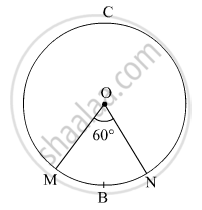Practice set 7.3 | Q 6.2 | Page 154
In the given figure, radius of the circle is 7 cm and m ( arc MBN) = 60°,
find  A(O - MBN) .Practice set 7.3 | Q 6.3 | Page 154
In the given figure, the radius of the circle is 7 cm and m ( arc MBN) = 60°,
find  A(O - MCN).Practice set 7.3 | Q 7 | Page 155

In the given figure, radius of circle is 3.4 cm and perimeter of sector P-ABC is 12.8 cm . Find A(P-ABC).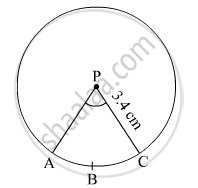Practice set 7.3 | Q 8 | Page 155

In the given figure, O is the centre of the sector. $\angle$ROQ =  $\angle$MON = 60° . OR = 7 cm, and   OM = 21 cm. Find the lengths of  arc RXQ and arc MYN. ($\pi = \frac{22}{7}$)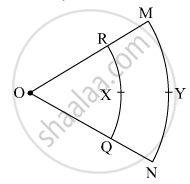Practice set 7.3 | Q 9 | Page 155

In the given figure, if A(P-ABC) = 154 cm2  radius of the circle is 14 cm, find

(1) ∠APC

(2) l ( arc ABC) .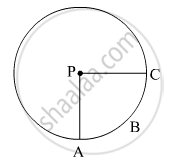Practice set 7.3 | Q 10.1 | Page 155
The radius of a sector of a circle is 7 cm. If the measure of the arc of the sector is - 30°  find the area of the sector in case.
Practice set 7.3 | Q 10.3 | Page 155

The radius of a sector of a circle is 7 cm. If the measure of the arc of the sector is three right angles, find the area of the sector in each case.

Practice set 7.3 | Q 10.3 | Page 155

The radius of a sector of a circle is 7 cm. If the measure of the arc of the sector is - three right angles; find the area of the sector in case.

Practice set 7.3 | Q 11 | Page 155

The area of a minor sector of a circle is 3.85 cmand the measure of its central angle is 36°. Find the radius of the circle.

Practice set 7.3 | Q 12 | Page 155

In the given figure,

$\square$ PQRS is a rectangle. If PQ = 14 cm, QR = 21 cm, find the areas of the parts x, y, and z.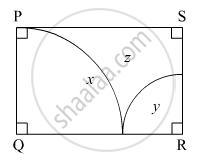Practice set 7.3 | Q 13.1 | Page 155
$∆$ LMN is an equilateral triangle. LM = 14 cm. As shown in the figure, three sectors are drawn with vertices as centers and radius 7 cm.
Find, A ( $∆$ LMN)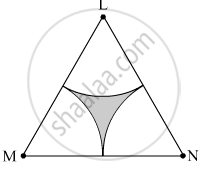Practice set 7.3 | Q 13.2 | Page 155
∆LMN is an equilateral triangle. LM = 14 cm. As shown in the figure, three sectors are drawn with vertices as centers and radius 7cm. Find, Area of any one of the sectors.Practice set 7.3 | Q 13.3 | Page 155
$∆$ LMN is an equilateral triangle. LM = 14 cm. As shown in the figure, three sectors are drawn with vertices as centers and radius 7 cm.
Find, Total area of all the three sectors.Practice set 7.3 | Q 13.4 | Page 155
$∆$ LMN is an equilateral triangle. LM = 14 cm. As shown in the figure, three sectors are drawn with vertices as centers and radius 7 cm.
Find, Area of the shaded region.Practice set 7.4 [Pages 159 - 160]

### Balbharati solutions for Maharashtra state board (SSC) 10th Standard Maths 2 Geometry Chapter 7 Mensuration Practice set 7.4 [Pages 159 - 160]

Practice set 7.4 | Q 1 | Page 159

In the given figure, A is the centre of the circle. $\angle$ABC = 45° and AC = 7 $\sqrt{2}$cm. Find the area of segment BXC.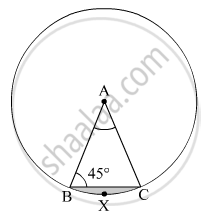Practice set 7.4 | Q 2 | Page 159

In the given figure, O is the centre of the circle. m ( arc PQR) = 60° OP = 10 cm. Find the area of the shaded region.( $\pi$= 3.14, $\sqrt{3}$= 1.73)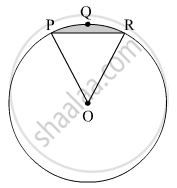Practice set 7.4 | Q 3 | Page 160

In the given figure, if A is the centre of the circle. $\angle$ PAR = 30°, AP = 7.5, find the area of the segment PQR ( $\pi$  = 3.14)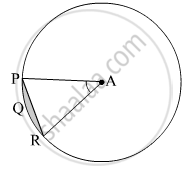Practice set 7.4 | Q 4 | Page 160

In the given figure, if O is the center of the circle, PQ is a chord. $\angle$ POQ = 90°, area of the shaded region is 114 cm2, find the radius of the circle. $\pi$ = 3.14)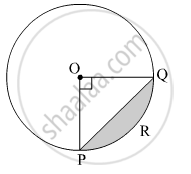Practice set 7.4 | Q 5 | Page 160

A chord PQ of a circle with a radius of cm subtends an angle of 60° with the center of the circle. Find the area of the minor as well as the major segment. ( $\pi$ = 3.14,  $\sqrt{3}$ = 1.73)

Practice set 7.4 [Pages 160 - 163]

### Balbharati solutions for Maharashtra state board (SSC) 10th Standard Maths 2 Geometry Chapter 7 Mensuration Practice set 7.4 [Pages 160 - 163]

Practice set 7.4 | Q 1.1 | Page 160
Choose the correct alternative answer for the following question.
The ratio of circumference and area of a circle is 2:7. Find its circumference.
• $14\pi$

•   $\frac{7}{\pi}$

• 7$\pi$

• $\frac{14}{\pi}$

Practice set 7.4 | Q 1.2 | Page 160
Choose the correct alternative answer for the following question.
If measure of an arc of a circle is 160° and its length is 44 cm, find the circumference of the circle.

• 66 cm

•  44 cm

•  160 cm

•  99 cm

Practice set 7.4 | Q 1.3 | Page 160

Choose the correct alternative answer for the following question.

Find the perimeter of a sector of a circle if its measure is 90° and radius is 7 cm.

•  44 cm

•   25 cm

• 36 cm

• 56 cm

Practice set 7.4 | Q 1.4 | Page 160

Choose the correct alternative answer for the following question.

Find the curved surface area of a cone of radius 7 cm and height 24 cm.
• 440 cm

• 550 cm2

• 330 cm

• 110 cm2

Practice set 7.4 | Q 1.5 | Page 160

Choose the correct alternative answer for the following question.

The curved surface area of a cylinder is 440 cm 2 and its radius is 5 cm. Find its height.

•  $\frac{44}{\pi}$ cm

• 22 $\pi$ CM

•  44 $\pi$ CM

• $\frac{22}{\pi}$ cm

Practice set 7.4 | Q 1.6 | Page 160

Choose the correct alternative answer for the following question.

A cone was melted and cast into a cylinder of the same radius as that of the base of the cone. If the height of the cylinder is 5 cm, find the height of the cone.

• 15 cm

• 10 cm

• 18 cm

•  5 cm

Practice set 7.4 | Q 1.7 | Page 160

Choose the correct alternative answer for the following question.

Find the volume of a cube of side 0.01 cm.
• 1 cm3

• 0.001 cm

• 0.0001 cm3

• 0.000001 cm3

Practice set 7.4 | Q 1.8 | Page 160

Choose the correct alternative answer for the following question.

Find the side of a cube of volume 1 m.
•  1 cm

• 10 cm

• 100 cm

• 1000 cm

Practice set 7.4 | Q 2 | Page 161

A washing tub in the shape of a frustum of a cone has a height of 21 cm. The radii of the circular top and bottom are 20 cm and 15 cm respectively. What is the capacity of the tub? ( $\pi = \frac{22}{7}$).

Practice set 7.4 | Q 3 | Page 161

Some plastic balls of radius 1 cm were melted and cast into a tube. The thickness, length and outer radius of the tube were 2 cm, 90 cm, and 30 cm respectively. How many balls were melted to make the tube?

Practice set 7.4 | Q 4 | Page 161

A metal parallelopiped of measures 16 cm x 11 cm x 10 cm was melted to make coins. How many coins were made if the thickness and diameter of each coin were 2 mm and 2 cm respectively?

Practice set 7.4 | Q 5 | Page 161

The diameter and length of a roller is 120 cm and 84 cm respectively. To level the ground, 200 rotations of the roller are required. Find the expenditure to level the ground at the rate of Rs. 10 per sq.m.

Practice set 7.4 | Q 6 | Page 161

The diameter and thickness of a hollow metals sphere are 12 cm and 0.01 m respectively. The density of the metal is 8.88 gm per cm3. Find the outer surface area and mass of the sphere.

Practice set 7.4 | Q 7 | Page 161

A cylindrical bucket of diameter 28 cm and a height of 20 cm was full of sand. When the sand in the bucket was poured on the ground, the sand got converted into a shape of a cone. If the height of the cone was 14 cm, what was the base area of the cone?

Practice set 7.4 | Q 8 | Page 161
The radius of a metallic sphere is 9 cm. It was melted to make a wire of diameter 4 mm. Find the length of the wire.
Practice set 7.4 | Q 9 | Page 161

The area of a sector of a circle of 6 cm radius is 15 $\pi$ sq.cm. Find the measure of the arc and length of the arc corresponding to the sector.

Practice set 7.4 | Q 10 | Page 161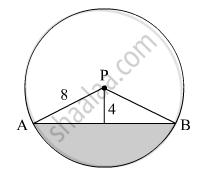In the given figure, seg AB is a chord of a circle with centre P. If PA = 8 cm and distance of chord AB from the centre P is 4 cm, find the area of the shaded portion. ( $\pi$ = 3.14, $\sqrt{3}$= 1.73 )

Practice set 7.4 | Q 11 | Page 162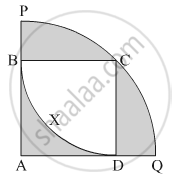In the given figure, square ABCD is inscribed in the sector A - PCQ. The radius of sector C - BXD is 20 cm. Complete the following activity to find the area of shaded region

Practice set 7.4 | Q 12 | Page 163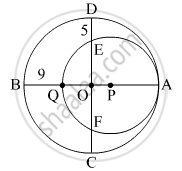In the given figure, two circles with centres O and P are touching internally at point A. If BQ = 9, DE = 5, complete the following activity to find the radii of the circles.

## Chapter 7: Mensuration

Practice set 7.1Practice set 7.2Practice set 7.3Practice set 7.4## Balbharati solutions for Maharashtra state board (SSC) 10th Standard Maths 2 Geometry chapter 7 - Mensuration

Balbharati solutions for Maharashtra state board (SSC) 10th Standard Maths 2 Geometry chapter 7 (Mensuration) include all questions with solution and detail explanation. This will clear students doubts about any question and improve application skills while preparing for board exams. The detailed, step-by-step solutions will help you understand the concepts better and clear your confusions, if any. Shaalaa.com has the Maharashtra State Board Maharashtra state board (SSC) 10th Standard Maths 2 Geometry solutions in a manner that help students grasp basic concepts better and faster.

Further, we at Shaalaa.com provide such solutions so that students can prepare for written exams. Balbharati textbook solutions can be a core help for self-study and acts as a perfect self-help guidance for students.

Concepts covered in Maharashtra state board (SSC) 10th Standard Maths 2 Geometry chapter 7 Mensuration are Conversion of Solid from One Shape to Another, Frustum of a Cone, Concept of Surface Area, Volume, and Capacity, Length of an Arc, Circumference of a Circle, Surface Area of a Combination of Solids, Euler's Formula, Areas of Sector and Segment of a Circle, Volume of a Combination of Solids, Circumference of a Circle.

Using Balbharati Class 10th Board Exam solutions Mensuration exercise by students are an easy way to prepare for the exams, as they involve solutions arranged chapter-wise also page wise. The questions involved in Balbharati Solutions are important questions that can be asked in the final exam. Maximum students of Maharashtra State Board Class 10th Board Exam prefer Balbharati Textbook Solutions to score more in exam.

Get the free view of chapter 7 Mensuration Class 10th Board Exam extra questions for Maharashtra state board (SSC) 10th Standard Maths 2 Geometry and can use Shaalaa.com to keep it handy for your exam preparation